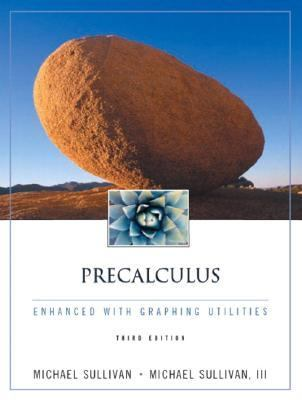214791

9780130659156

# Precalculus Enhanced With Graphing Utilities Enhanced With Graphing Utilities\$86.87
\$3.95 Shipping
List Price
\$114.00
Discount
23% Off
You Save
\$27.13

• Condition: New
• Provider: Ergodebooks Contact
• Provider Rating:
82%
• Ships From: Multiple Locations
• Shipping: Standard
• Comments: Buy with confidence. Excellent Customer Service & Return policy. Ships Fast. 24*7 Customer Service.30-day money back guarantee
\$0.51
\$3.95 Shipping
List Price
\$114.00
Discount
99% Off
You Save
\$113.49

• Condition: Very Good
• Provider: HippoBooks Contact
• Provider Rating:
86%
• Ships From: Multiple Locations
• Shipping: Standard
• Comments: Light rubbing wear to cover, spine and page edges. Very minimal writing or notations in margins not affecting the text. Possible clean ex-library copy, with their stickers and or stamp(s).30-day money back guarantee

Most renters respond to questions in 48 hours or less.
The response will be emailed to you.
• ISBN-13: 9780130659156
• ISBN: 0130659150
• Edition: 3
• Publication Date: 2002
• Publisher: Prentice Hall PTR

### AUTHOR

Sullivan, Michael

### SUMMARY

(NOTE: Each chapter concludes with Chapter Review.) 1. Graphs. Rectangular Coordinates; Graphing Utilities. Introduction to Graphing Equations. Symmetry; Graphing Key Equations; Circles. Solving Equations Using a Graphing Utility. Solving Inequalities. Lines. Chapter Projects. Cumulative Review. 2. Functions and Their Graphs. Functions. Linear Functions and Models. Properties of Functions. Library of Functions; Piecewise-Defined Functions. Graphing Techniques: Transformations. Operations on Functions; Composite Functions. Mathematical Models: Constructing Functions. Chapter Projects. Cumulative Review. 3. Polynomial and Rational Functions. Quadratic Functions and Models. Power Functions and Models. Polynomial Functions and Models. Rational Functions I. Rational Functions II: Analyzing Graphs. Polynomial and Rational Inequalities. The Real Zeros of a Polynomial Function. Complex Zeros; Fundamental Theorem of Algebra. Chapter Projects. Cumulative Review. 4. Exponential and Logarithmic Functions. One-to-One Functions; Inverse Functions. Exponential Functions. Logarithmic Functions. Properties of Logarithms. Logarithmic and Exponential Equations. Compound Interest. Growth and Decay. Exponential, Logarithmic, and Logistic Curve Fitting. Chapter Projects. Cumulative Review. 5. Trigonometric Functions. Angles and Their Measure. Trigonometric Functions: Unit Circle Approach. Properties of the Trigonometric Functions. Graphs of the Sine and Cosine Functions. Graphs of the Tangent, Cotangent, Secant, and Cosecant Functions. Phase Shifts; Sinusoidal Curve Fitting. Chapter Projects. Cumulative Review. 6. Analytic Trigonometry. The Inverse Sine, Cosine, and Tangent Functions. The Inverse Trigonometric Functions (continued) Trigonometric Identities. Sum and Difference Formulas. Double-angle and Half-angle Formulas. Product-to-Sum and Sum-to-Product Formulas. Trigonometric Equations II. Trigonometric Equations II Chapter Review. Chapter Projects. Cumulative Review. 7. Applications of Trigonometric Functions. Right Triangle Trigonometry. Law of Sines. Law of Cosines. Area of a Triangle. Simple Harmonic Motion; Damped Motion; Combining Waves. Chapter Projects. Cumulative Review. 8. Polar Coordinates; Vectors. Polar Coordinates. Polar Equations and Graphs. The Complex Plane; DeMoivre''s Theorem. Vectors. The Dot Product. Vectors in Space. The Cross Product. Chapter Projects. Cumulative Review. 9. Analytic Geometry. Conics. The Parabola. The Ellipse. The Hyperbola. Rotation of Axes; General Form of a Conic. Polar Equations of Conics. Plane Curves and Parametric Equations. Chapter Projects. Cumulative Review. 10. Systems of Equations and Inequalities. Systems of Linear Equations: Two Equations Containing Two Variables. Systems of Linear Equations: Three Equations Containing Three Variables. Systems of Linear Equations: Matrices. Systems of Linear Equations: Determinants. Matrix Algebra. Partial Fraction Decomposition. Systems of Nonlinear Equations. Systems of Inequalities. Linear Programming. Chapter Projects. Cumulative Review. 11. Sequences, Induction, the Binomial Theorem. Sequences. Arithmetic Sequences. Geometric Sequences; Geometric Series. Mathematical Induction. The Binomial Theorem. Chapter Projects. Cumulative Review. 12. Counting and Probability. Sets and Counting. Permutations and Combinations. Probability of Equally Likely Outcomes. Obtaining Probabilities from Data. Chapter Projects. Cumulative Review. 13. A Preview of Calculus: The Limit, Derivative, and Integral of a Function. Finding Limits Using Tables and Graphs. Algebra Techniques for Finding Limits. One-sided Limits; Continuous Functions. The Tangent Problem; the Derivative. The Area Problem; the Integral. Chapter Projects. Cumulative Review. Appendix Review. Algebra Review. Geometry Review. Polynomials. Rational Expressions. Polynomial Division; Synthetic Division. Equations. Complex Numbers; Quadratic Equations with a Negative Discriminant. Setting up Equations; Applications. nth Roots; Rational Exponents. Answers. Index.Sullivan, Michael is the author of 'Precalculus Enhanced With Graphing Utilities Enhanced With Graphing Utilities', published 2002 under ISBN 9780130659156 and ISBN 0130659150.

You can find lots of answers to common customer questions in our FAQs

View a detailed breakdown of our shipping prices